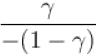Courses

# Maxwell's Relations MCQ Level – 2

## 10 Questions MCQ Test Topic wise Tests for IIT JAM Physics | Maxwell's Relations MCQ Level – 2

Description
This mock test of Maxwell's Relations MCQ Level – 2 for Physics helps you for every Physics entrance exam. This contains 10 Multiple Choice Questions for Physics Maxwell's Relations MCQ Level – 2 (mcq) to study with solutions a complete question bank. The solved questions answers in this Maxwell's Relations MCQ Level – 2 quiz give you a good mix of easy questions and tough questions. Physics students definitely take this Maxwell's Relations MCQ Level – 2 exercise for a better result in the exam. You can find other Maxwell's Relations MCQ Level – 2 extra questions, long questions & short questions for Physics on EduRev as well by searching above.
QUESTION: 1

### Ratio of the adiabatic coefficient of expansion which is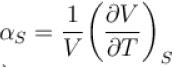to the isobaric coefficient of expansion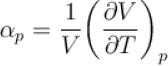is Select one:

Solution: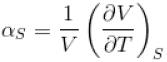Isobaric volume coefficient of expansion,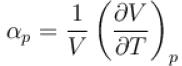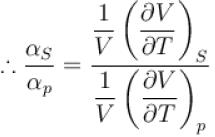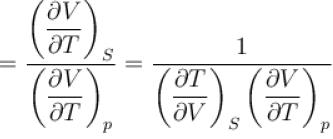But, from Maxwell's relation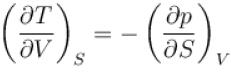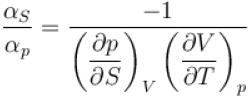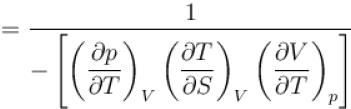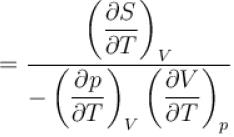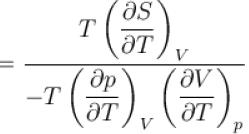But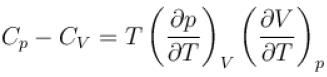and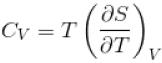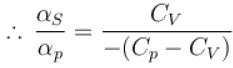or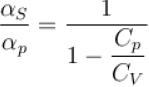or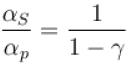The correct answer is: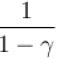QUESTION: 2

### For a perfect gas Joule - Thomson cofficient µ is equal to Select one:

Solution:

Joule - Thomson coefficient µ,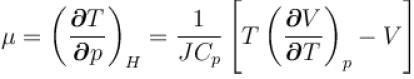For 1 mole of perfect gas, the equation of state is pV = RT
Differentiating it with respect to T, taking P constant,
We have,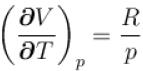or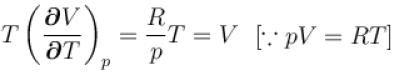or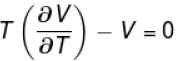Substituting this result in equation (1) we get,
Joule-Thomson coefficient, μ = 0

QUESTION: 3

### Calculate under what pressure (atm) water would boil at 150°C. If the change in specific volume when 1 gram of water is converted into steam is 1676cc. Given, latent heat of vaporization of steam = 540 cal/g; J = 4.2×107 erg/cal and one atmosphere pressure = 106 dynes/cm2 Select one:

Solution:

Here, ∂H = 540 cal.
= 540 × 4.2 × 107 erg.
∂V = 1676cc
Boiling Temperature T = 373 K
Temperature when water is to be boil = 150°C
= 150 + 273 = 423 K.
∂T = 423 – 373 = 50 K
∂p = ?
Applying these value in the maxwell's thermodynamical relation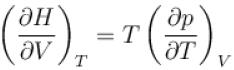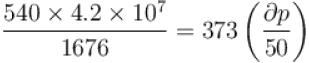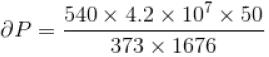= 1.814 × 106 dynes/cm2
= 1.814 atmosphere
Therefore, the pressure at which water would boil at 150°C
= 1.814 + 1,000 = 2.814 atmospheric pressure.

QUESTION: 4

The normal boiling point of benzene is 80°C. The latent heat of vaporization is 380 Joule/g, density of vapour at boiling points is 4g/litre and that of the liquid 0.9g/cm3 then the boiling point of benzene under a pressure of 80 cm of mercury.
Select one:

Solution:

Here, change in pressure, dP = 80 – 76
= 4 cm of Hg
= 4 × 13.6 × 980 dynes/cm2
Normal Boiling point, T = 80°C]
= 80 + 273 = 353 K
L = 980 joule/g
= 380 × 107 erg/g.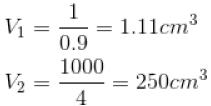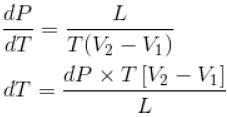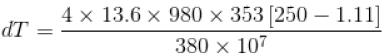= 1.233 K
=1.233°C
The boiling point of benzene at a pressure of 80 cm of Hg
= 80 + 1.233
= 81.233°C

QUESTION: 5

For the perfect gas,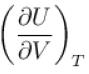is equal to
Select one:

Solution:

From the maxwell's relation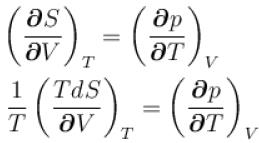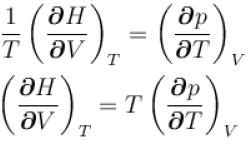But dH = dU + pdV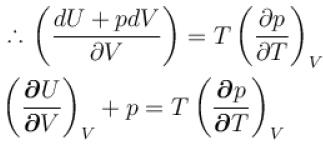or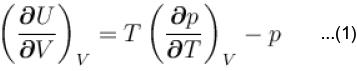But from perfect gas equation
pV = RT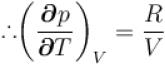Putting in (1)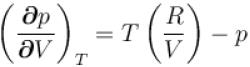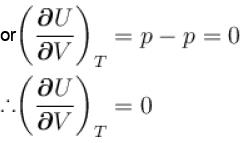QUESTION: 6

For any substance, the ratio of adiabatic and isothermal elasticities which are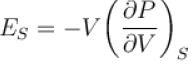and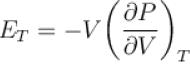respectively is equal to :
Select one:

Solution:

Isothermal elasticity,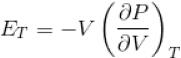Adiabatic elasticity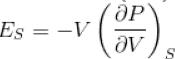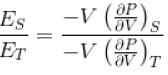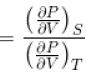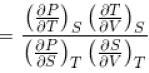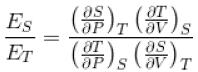But from the first four Maxwell's equation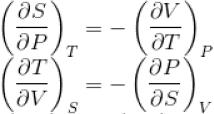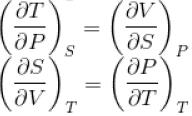∴ Substituting these values in equation (1)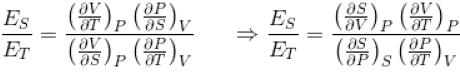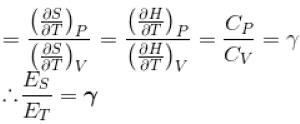The correct answer is: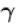QUESTION: 7

If C1 and C2 represents the specific heat of a liquid and its saturated vapour respectively and L is the latent heat of the vapour then clausius latent heat equation is given by :
Select one:

Solution:

For a change of state from liquid to vapour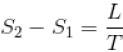...(i)
Here S1 and S2 are the entropies in the liquid and vapour states respectively Differentiating equation (1) with respect to T.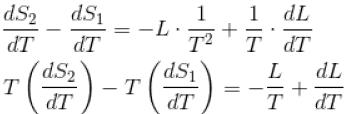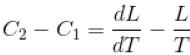This equation is known as clausius latent heat equation.
The correct answer is: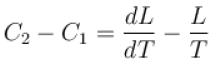QUESTION: 8

The Gibb’s function G in thermodynamics is defined as
G = H – TS
(where, H = Enthalpy, T = Temperature, S = Entropy)
In an isothermal, isobaric, reversible process, G
Select one:

Solution:

Condition for a change to occur in any processes
dU – TdS ≤ pdV
d(U + pV – TS) ≤ 0
d(H – TS) ≤ 0
as [H = U + pV]
G = H – TS
or dG ≤ 0
It means is isothermal, Isobaric and reversible process, dG = 0 or G = constant
The correct answer is: remains constant but not zero

QUESTION: 9

The thermodynamical relation expressing TdS equation
Select one:

Solution:

Let the entropy S of a thermodynamic system be a function of temperature T and volume v, i.e. S = f(T, V)
Since, dS is perfect differential, we can write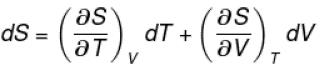Multiplying by T, we get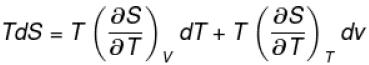For any substance, the specific heat at constant volume is given by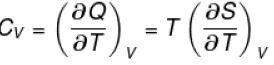Using dQ = TdS
Also from Maxwell's equation, we have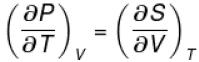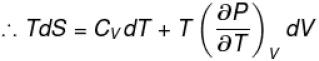The correct answer is: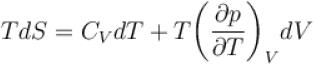QUESTION: 10

The ratio of the adiabatic to the isochoric pressure coefficient of expansion is
Select one:

Solution: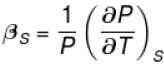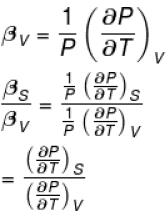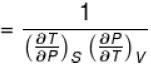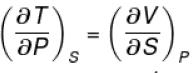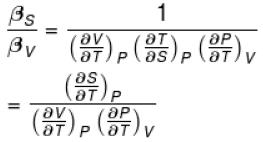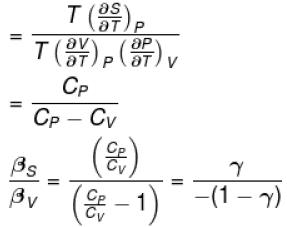The correct answer is: